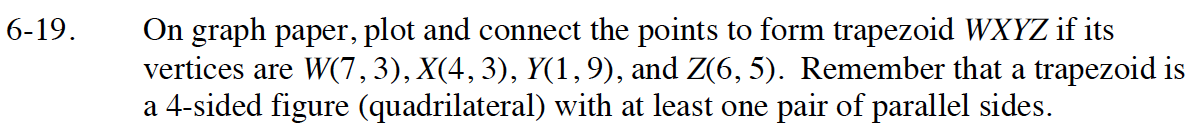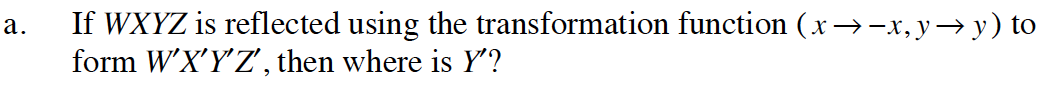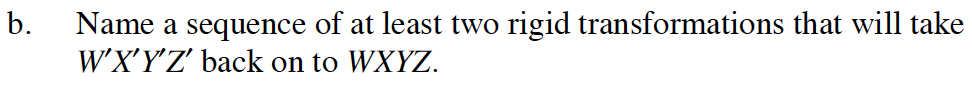Home > GB8I > Chapter cc46 > Lesson cc46.1.2 > Problem6-19

6-19.
1. On graph paper, plot and connect the points to form trapezoid WXYZ if its vertices are W(7, 3), X(4, 3), Y(1, 9), and Z(6, 5). Remember that a trapezoid is a 4-sided figure (quadrilateral) with at least one pair of parallel sides. 6-19 HW eTool (Desmos). Homework Help ✎

1. If WXYZ is reflected using the transformation function (x → −x, yy) to form WXYZ′, then where is Y′?

2. Name a sequence of at least two rigid transformations that will take WXYZ′ back on to WXYZ.Transform each point according to the transformation function. For W this would be: (7 → −7, 3 → 3) so W ' becomes (−7, 3).Use the eTool to explore various transformations for part (b).
Click on the link at the right for the full eTool version: INT1 6-19 HW eTool